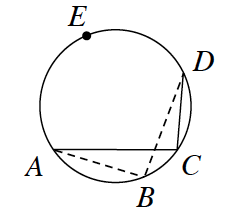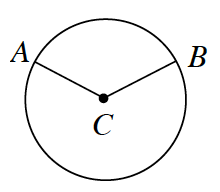### Home > CCG > Chapter Ch10 > Lesson 10.2.2 > Problem10-87

10-87.

Review what you know about the angles and arcs of circles below.

1. A circle is divided into nine congruent sectors. What is the measure of each central angle?

What is the total angle measure of a circle?

1. In the diagram below, find $m\overarc { A E D }$ and $m∠C$ if $m∠B = 97º$.

How are inscribed angles related to intercepted arcs?

$m\overarc{AED} = 2(97º) = 194º$

$m\angle {C}= 0.5(194º)=97º$1. In $⊙C$ below, $m∠ACB = 125º$ and $r = 8$ inches. Find $m\overarc{ A B }$ and the length of $\overarc{ A B }$. Then find the area of the smaller sector.

• How does the angle measure relate to the arc measure?

Intercepted arcs of equal measure to their corresponding central angles.

$\overparen{AB}=\angle {ACB}=125º$

Circumference of the Whole Circle $= 2πr = 2π(8) = 16π$

Area of Circle $= πr^2 = π(8)^2 = 64π$

$m\overarc{AB}= \frac {125º}{360º}\cdot 16\pi = \frac{ 2000}{360} \pi ≈ 17.5 \text{ in}$

$\text{Area of Sector}=\frac{125º}{360º}\cdot64\pi=\frac{8000}{360}\pi=69.8\text{ in}^2$